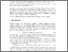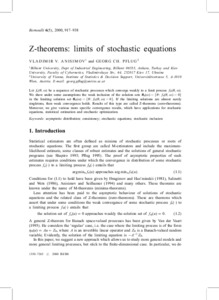# Z-theorems: Limits of stochastic equations

Anisimov, A. & (2000). Z-theorems: Limits of stochastic equations. Bernoulli 6 (5) 917-938.Preview Text Z-theorems limits of stochastic equations.pdf - Published Version Available under License Creative Commons Attribution. Download (378kB) | Preview

## Abstract

Let fn(θ,ω) be a sequence of stochastic processes which converge weakly to a limit process f0(θ,ω). We show under some assumptions the weak inclusion of the solution sets θn(ω)={θ:fn(θ,ω)=0} in the limiting solution set θ0(ω)={θ:f0(θ,ω)=0} . If the limiting solutions are almost surely singletons, then weak convergence holds. Results of this type are called Z-theorems (zero-theorems). Moreover, we give various more specific convergence results, which have applications for stochastic equations, statistical estimation and stochastic optimization.

Item Type: Article Risk, Modeling and Society (RMS) IIASA Import 15 Jan 2016 02:11 27 Aug 2021 17:16 https://pure.iiasa.ac.at/5986

### Actions (login required)View Item